Open in App
Not now

# Doubly Linked List using Sentinel Nodes

• Difficulty Level : Hard
• Last Updated : 22 Feb, 2023

In the case of the simple doubly linked list, if we have to perform the insertion or deletion operation at the starting of the doubly linked list, end of the doubly linked list, or in between the starting and end nodes for each, we require to check different condition that makes the algorithm complex so to solve this problem we can use doubly linked list along with the sentinel nodes.

## What is a sentinel node?

Sentinel nodes are specially designed nodes that do not hold or refer to any data of the doubly linked list (that data structure).

To make insertion and deletion operations in a doubly-linked list simple, we have to add one sentinel node at the beginning of the doubly linked list and one at the end of the doubly linked list, as shown in the below diagram.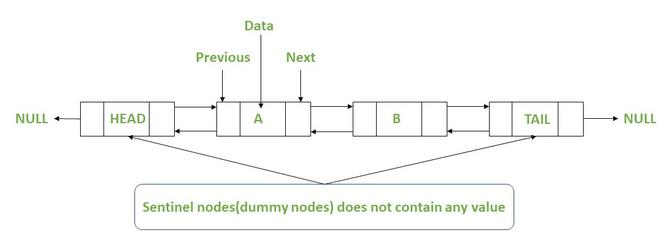Doubly linked list with sentinel nodes

As shown above, each node other than the sentinel nodes contains the pointer to the previous and next node along with data.

The most significant advantage of using the sentinel nodes in the doubly linked list:

• By adding the sentinel nodes to the doubly linked list now for the deletion or insertion at the beginning, end or in between the beginning and end nodes of the linked list, we do not need to write the different conditions for each one.
• All these operations can be done as the deletion or insertion between the beginning and end node of a doubly linked list.

## Structure of a Doubly-Linked List using sentinel nodes:

The structure of each node and the creation of the new node in a doubly-linked list with sentinel nodes are the same as the simple doubly-linked list, as shown below.

## C++

 `// Each node contains the data,` `// previous node pointer and` `// the next node pointer` `struct` `node {` `    ``int` `data;` `    ``struct` `node* next;` `    ``struct` `node* pre;` `};`   `// To create a node, first define the node pointer` `// and than assign the memory required by the node` `// and return the node` `struct` `node* createnode()` `{` `    ``struct` `node* t;` `    ``t = (``struct` `node*)``malloc``(``sizeof``(``struct` `node));` `    ``return` `(t);` `}`

## Java

 `// Each node contains the data,` `// previous node pointer and` `// the next node pointer` `static` `class` `Node {` `    ``int` `data;` `     ``node next;` `     ``node pre;` `}`   `// To create a node, first define the node pointer` `// and than assign the memory required by the node` `// and return the pointer` `Node createnode()` `{` `    ``Node node = ``new` `Node();` ` ``retutn node; ` `}`

## Python

 `# Each node contains the data,` `# previous node pointer and` `# the next node pointer` `class` `Node:` `    ``def` `__init__(``self``, data):` `        ``self``.data ``=` `data` `        ``self``.``next` `=` `None` `        ``self``.pre ``=` `None`   `# To create a node, first define the node pointer` `# and than assign the memory required by the node` `# and return the pointer` `def` `createNode():` `    ``return` `Node(``None``)`

## C#

 `// Each node contains the data,` `// previous node pointer and` `// the next node pointer` `public` `static` `class` `Node {` `    ``public` `int` `data;` `    ``public` `Node next;` `    ``public` `Node pre;` `}`   `// To create a node, first define the node pointer` `// and than assign the memory required by the node` `// and return the pointer` `public` `static` `Node CreateNode()` `{` `    ``Node node = ``new` `Node();` `    ``return` `node;` `}`   `// This code is contributed by akashish__`

## Javascript

 `// Each node contains the data,` `// previous node pointer and` `// the next node pointer` `class Node {` `    ``constructor(data) {` `        ``this``.data = data;` `        ``this``.next = ``null``;` `        ``this``.pre = ``null``;` `    ``}` `}`   `// To create a node, first define the node pointer` `// and than assign the memory required by the node` `// and return the node` `function` `createNode() {` `    ``return` `new` `Node();` `}`

## Operations on a doubly-linked list using Sentinel Nodes:

The common operations in a doubly-linked list are:

1. Insert new node
2. Delete existing node
3. Display all the nodes’ data

### 1. Insert a new node in a Doubly-Linked List using Sentinel Nodes

Insertion of a new node in a doubly-linked list can be done in three ways, but as we have used the sentinel node in the doubly linked list, so we do not have to write a different algorithm for each case we have to give the location of the node after which we have to add the new node to the linked list as shown in the code.

• Insert a new node at the beginning of the doubly-linked list
In this, we are adding the new node just after the head sentinel node. So basically, we are adding the new node at the beginning of the doubly linked list. Still, it will behave as the addition of a node between the beginning and end node of the linked list due to the sentinel node.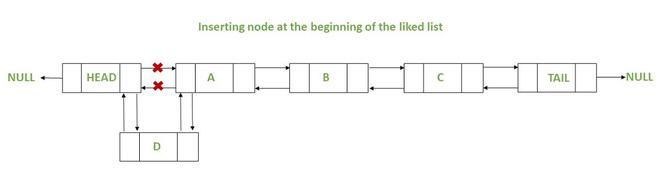Insertion of node at beginning of the doubly linked list

• Insert a new node at a given position in the linked list
As shown in the given below diagram, we are adding a new node in the middle of the linked list.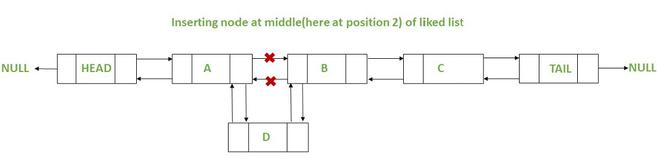Insertion of node at a given position in the doubly linked list

• Insert a new node at the end of the doubly linked list
In this, we are adding the new node just before the tail sentinel node. So basically, we are adding the new node at the end of the doubly linked list. Still, it will behave as the addition of a node in between the beginning and end node of the linked list due to the sentinel node.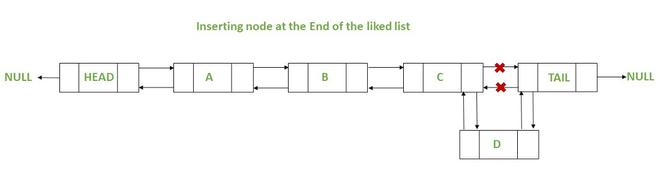Insertion of node at end of the doubly linked list

### 2. Delete an existing node in a Doubly-Linked List using Sentinel Nodes

Deleting an existing node in a doubly-linked list can also be done in three ways. Still, as we have used the sentinel node in the doubly linked list, so we do not have to write a different algorithm for each case, we have to pass the location of the node, which we want to delete.

• Delete an existing node at the beginning of the doubly linked list
In this, we are deleting an existing node just after the head sentinel node. So basically, we are deleting an existing node at the beginning of the doubly linked list. Still, it will behave as the deletion of a node between the beginning and end node of the linked list due to the sentinel node.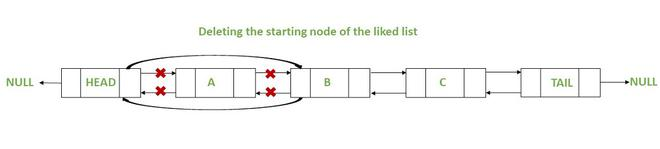Deleting the starting node in a doubly-linked list

• Delete an existing node at a given position in the linked list
As shown in the diagram given below, we are deleting an existing node at a given location of the linked list.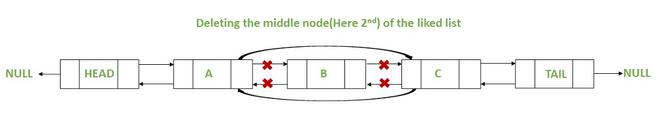Deleting node at a given position in the doubly linked list

• Delete an existing node at the end of the doubly linked list
In this, we are deleting an existing node just before the tail sentinel node. So basically, we are deleting an existing node at the end of the doubly linked list. Still, it will behave as the deletion of a node between the beginning and end node of the linked list due to the sentinel node.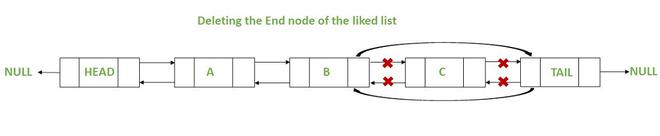Deleting the ending node in the doubly linked list

### 3. Display all the nodes’ data in a Doubly-Linked List using Sentinel Nodes

It is the same as the simple doubly linked list. We have to travel through all the nodes and print the data stored in them except the sentinel nodes.

Implementation: The full implementation of the doubly linked list with sentinel nodes and insertion, deletion, and display operation is shown below.

## C++

 `// C++ code to implement doubly-linked list` `// using sentinel node`   `#include ` `using` `namespace` `std;`   `int` `size = 0;`   `// Each node contains the data,` `// previous node pointer and` `// the next node pointer` `struct` `node {` `    ``int` `data;` `    ``struct` `node* next;` `    ``struct` `node* pre;` `};`   `// To create a node, first define the node pointer` `// and than assign the memory required by node` `// and return the pointer` `struct` `node* createnode()` `{` `    ``struct` `node* t;` `    ``t = (``struct` `node*)``malloc``(``sizeof``(``struct` `node));` `    ``return` `(t);` `}`   `// Function to display all the nodes` `void` `display(``struct` `node* head,` `             ``struct` `node* tail)` `{` `    ``head = head->next;` `    ``cout << ``"\nThe linked list is :-  "``;` `    ``while` `(head != tail) {` `        ``cout << head->data << ``" "``;` `        ``head = head->next;` `    ``}` `}`   `// Function to insert a new node` `void` `insertion(``struct` `node* head,` `               ``struct` `node* tail,` `               ``int` `n, ``int` `value)` `{` `    ``n--;` `    ``struct` `node *temp, *ptr;` `    ``ptr = head;` `    ``temp = createnode();` `    ``temp->data = value;`   `    ``int` `i = 0;`   `    ``// Run's until reach the node after` `    ``// which we have to add the new node` `    ``while` `(i < n) {` `        ``ptr = ptr->next;` `        ``i++;` `    ``}` `    ``temp->next = ptr->next;` `    ``temp->pre = ptr;` `    ``ptr->next = temp;` `    ``(temp->next)->pre = temp;`   `    ``// Linked list size is increased by 1` `    ``size++;` `}`   `// Function to delete an element` `// from the doubly-linked list` `void` `deletion(``struct` `node* head,` `              ``struct` `node* tail, ``int` `n)` `{` `    ``n--;`   `    ``// If linked list is empty` `    ``if` `(head->next == tail) {` `        ``cout << ``"\nerror : linked list is empty"``;` `        ``return``;` `    ``}`   `    ``// If entered position is more` `    ``// than the size of the linked list` `    ``if` `(n >= size) {` `        ``cout << ``"\nerror : position is larger"` `                ``" than size of linked list"``;` `        ``return``;` `    ``}` `    ``struct` `node* ptr = head;` `    ``struct` `node* temp;` `    ``int` `i = 0;`   `    ``// Run's until reach the node whose` `    ``// next node have to deleted` `    ``while` `(i < n) {` `        ``ptr = ptr->next;` `        ``i++;` `    ``}`   `    ``cout << ``"\nDeleting node at position "` `         ``<< n + 1 << ``" contains value "` `         ``<< (ptr->next)->data;` `    ``temp = (ptr->next)->next;` `    ``ptr->next = temp;` `    ``temp->pre = ptr;`   `    ``// Size of the linked list decreased by 1` `    ``size--;` `}`   `// Driver code` `int` `main()` `{` `    ``// Here we are creating two sentinel nodes` `    ``// (does not contain any data)` `    ``struct` `node* head = createnode();` `    ``head->pre = NULL;`   `    ``struct` `node* tail = createnode();` `    ``tail->pre = head;` `    ``tail->next = NULL;`   `    ``head->next = tail;`   `    ``int` `n;`   `// Declaring start position of goto section` `start:` `    ``cout << ``"\n1. Insertion\n2. Deletion\n"` `            ``"3. Display\n0. Exit\n"``;` `    ``cin >> n;` `    ``switch` `(n) {` `    ``case` `1:`   `        ``// Insertion at the beginning` `        ``// of the Doubly linked list` `        ``insertion(head, tail, 1, 10);` `        ``display(head, tail);`   `        ``// Insertion at the End` `        ``// of the Doubly linked list` `        ``insertion(head, tail, size + 1, 14);` `        ``display(head, tail);`   `        ``// Inserting node in between` `        ``// the doubly linked list` `        ``insertion(head, tail, 2, 8);` `        ``display(head, tail);` `        ``cout << endl;` `        ``goto` `start;` `        ``break``;`   `    ``case` `2:`   `        ``// Deleting the node at location 2` `        ``deletion(head, tail,` `                 ``2);` `        ``display(head, tail);` `        ``cout << endl;`   `        ``// Deleting the first node` `        ``deletion(head, tail, 1);` `        ``display(head, tail);` `        ``cout << endl;`   `        ``// Deleting the last node` `        ``deletion(head, tail, size);` `        ``display(head, tail);` `        ``cout << endl;` `        ``goto` `start;`   `    ``case` `3:` `        ``display(head, tail);` `        ``cout << endl;` `        ``goto` `start;`   `    ``default``:` `        ``break``;` `    ``}`   `    ``return` `0;` `}`

Output:

```1.Insertion
2.Deletion
3.Display
0.Exit
1

The linked list is :-  10
The linked list is :-  10 14
The linked list is :-  10 8 14

1.Insertion
2.Deletion
3.Display
0.Exit
3

The linked list is :-  10 8 14

1.Insertion
2.Deletion
3.Display
0.Exit
2

Deleting node at position 2 contains value 8
The linked list is :-  10 14

Deleting node at position 1 contains value 10
The linked list is :-  14

Deleting node at position 1 contains value 14